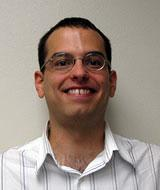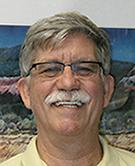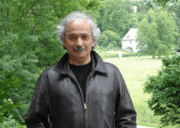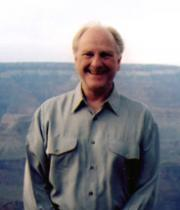# All Research### Matthew Blair

Pure Mathematics

My research deals with harmonic analysis and its interactions with partial differential equations of wave and Schroedinger type.  Much of my work involves finding effective ways to represent solutions to these equations and understanding the oscillatory integrals that appear as a result.  In particular, I am interested in the development of regularity and norm estimates for solutions that can be obtained this way.  This includes Strichartz, local smoothing, and squarefunction inequalities, which are all families of space-time integrability (L^p) estimates. They are important for various nonlinear equations and can also have applications to eigenfunction problems.

Most recently, my work has focused on establishing these inequalities for solutions over domains with a boundary.  Here the boundary conditions can influence the development of waves and affect the flow of energy. Understanding this phenomena often involves a connection with the field of microlocal analysis.  Here one studies waves by carefully localizing them both in space and in direction of propagation.  One effective method in this direction is to represent waves as superpositions of "wave packets", approximate solutions which are highly concentrated in both space and in frequency.  Wave packet methods continue to be influenced by ideas from both microlocal and harmonic analysis.### Charles Boyer

Pure Mathematics

My research interests range over a fairly broad part of modern differential geometry. It interfaces with the theory of Lie groups and both algebraic geometry and algebraic topology. Recently there have been two main threads. The first involves the study of the topology of moduli spaces of holomorphic maps, the so-called sigma-models, and of the moduli spaces of instantons.

The second uses contact geometry together with Lie group theoretical methods to give explicit constructions of Einstein manifolds of positive scalar curvature, and then to study the topology of such manifolds. Very recent work uses methods of algebraic geometry, more specifically Mori theory, to construct Einstein metrics on 5-manifolds, as well as homotopy spheres in all odd dimensions. This work appears in my monograph Sasakian Geometry with K. Galicki published by Oxford University Press

My earlier work was also two-tiered. The first involved group representation theory applied to problems of Mathematical Physics, in particular, to the problem of separation of variables of the partial differential equations that appear in Mathematical Physics. The second used Lie group methods to study complex geometry with special applications to general relativity.### Alexandru Buium

Pure Mathematics

I am interested in number theory and algebraic geometry. My recent work is devoted to establishing an arithmetic analogue of the theory of (ordinary/partial) differential equations. In the (ordinary) arithmetic theory the ``independent time variable'' t is replaced by a fixed prime integer p. Smooth real functions, x(t), are replaced by integer numbers, a, or, more generally, by integers in various (completions of) number fields.

The derivative operator on functions x -> dx/dt is replaced by a "Fermat quotient operator'' d which, on integer numbers, acts as da:=(a-a^p)/p. For details see my research monograph ``Arithmetic Differential Equations", Math. Surveys and Monographs 118, AMS, 2005.### Jehanzeb Chaudhry

Applied Mathematics

I design and analyse numerical methods for multi-physics, multi-rate partial differential equations. In particular, I focus on Adjoint based A posteriori error estimation to quantify the error in a quantity of a interest in a numerical solution. My recent interests include parallel-in-time integration, uncertainty quantification on stochastically perturbed domains, reduced order modeling and IMEX schemes for hyperbolic and parabolic problems. Another of my research areas is the numerical solution of PDEs using Least-Squares finite element methods, especially when the flux variables need to be approximated accurately. During my PhD thesis I looked at implicit solvation models for molecular dynamics and I still work in this field.

For more information, see my personal webpage.

Statistics

### Ronald Christensen

Statistics

Professor Christensen's research interests are in models that employ linear mean structures and in Bayesian methodology.  He contends that this includes virtually all of statistics.

### James Degnan

Statistics

My research focuses on statistical applications in genetics and evolutionary biology. Particular areas have included coalescent theory and its applications, phylogenetics, and human genetics.### James Ellison

Applied Mathematics

I'm an Applied Mathematician actively involved in Dynamical Systems, Probabilistic Methods, Stochastic Processes, Perturbation Theory, and Scientific Computing. I have worked on both fundamental and formal aspects of averaging methods in both deterministic and stochastic dynamical systems, but always with an eye for proofs of asymptotic validity.  Our main current focus is on problems defined by mean field Vlasov, Vlasov Fokker-Planck and Vlasov-Maxwell systems. The applications I have worked on are related to Beam Dynamics in Modern Particle Accelerators and Particle Channeling in Crystals .

For example, we are investigating coherent radiation and space charge in the context of charged particle bunches evolving under the mean field Vlasov-Maxwell dynamics.  This involves both analytic and numerical (e.g., meshless) approaches and the development of fast numerical algorithms in a high performance computing environment. In addition, we are beginning a study of the probabilistic Large Deviation Theory as a tool to study large N particle dynamics and the above mean field approximations.### Erik Erhardt

Statistics
A theme of my research is the development of statistical models motivated by real-world problems to reveal scientifically meaningful structure in multivariate data by decomposing a mixture into its constituent sources. Method development is based on parsimonious models which can extend to uncover more complicated structure in the data. This strategy allows a researcher to either address elemental, pre-specified scientific questions or reveal more complicated structure given enough evidence. Two areas of application are in estimating animal diets in ecological foodwebs and in using functional imaging of the human brain to estimate how connections between brain regions differ between patient populations. Implications include better evidence-based decisions for environmental management and improved understanding of mental differences and treatment.

(updated 20160107)

### Gabriel Huerta

Statistics

Gabriel Huerta's research underlies statistical modeling, computation and data analysis that has stemmed from a variety of collaborations.  He has strong interests in Bayesian Analysis, Time Series, Spatial-temporal modeling, stochastic simulation and computation. In particular, he developed a class of prior distributions for time series decompositions known as "structured AR priors" and that among other things, addresses issues of model order uncertainty and unitary roots.   In addition, Prof. Huerta is interested in space-time Dynamic Linear models for environmental and climatological data applications.   Within this framework, Prof. Huerta is  interested in hierarchical modeling to understand time-varying and spatial aspects for extreme value analysis.  Funded through NSF and DOE grants, he has collaborated with the Institute of Geophysics at the University of Texas-Austin in problems of Bayesian inversion for the calibration of the NCAR Community Atmosphere Model (CAM).